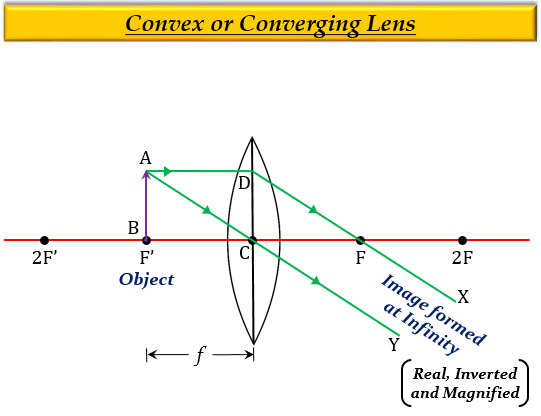# A magnifying lens has a focal length of 100 mm. An object whose size is 16 mm is placed at some distance from the lens so that an image is formed at a distance of 25 cm in front of the lens.(a) What is the distance between the object and the lens?(b) Where should the object be placed if the image is to form at infinity?

(a) Given:

Focal length of the lens $f$ = 100 mm

Height of object, $h$ = 16 mm

Image distance, $v$ = $-$25 cm = $-$250 mm      (negative because the image is virtual)

To find: Distance between the object and the lens, $u$.

Solution:

From the lens formula we know that-

$\frac {1}{v}-\frac{1}{u}=\frac{1}{f}$

Substituting the given values in the formula we get-

$\frac {1}{(-250)}-\frac{1}{u}=\frac{1}{100}$

$-\frac {1}{250}-\frac{1}{100}=\frac{1}{u}$

$\frac{1}{u}=\frac {-2-5}{500}$

$\frac{1}{u}=-\frac {7}{500}$

$u=-\frac {500}{7}$

$u=-71.4mm$

$u=-7.14cm$

Thus, the distance between the object and the lens is 7.14 cm.

(b) The object should be placed at the focus $F'$ (here 100mm) so that the image is formed at infinity.

So, $u$= $-$100mm = $-$10 cm.

Thus, the object should be placed at 10 cm in front of the lens.

Image is posted for reference onlyUpdated on: 10-Oct-2022

221 Views